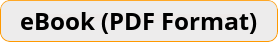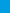# Grade 5 Common Core Mathematics Workbook 2018-2019 A Comprehensive Review and Step-by-Step Guide to Preparing for the Common Core Math TestGrade 5 Common Core Workbook is full of specific and detailed material that will be key to succeeding on the COMMON CORE Math. It’s filled with the critical math concepts a student will need in order to do well on the test. Math concepts in this book break down the topics, so the material can be quickly grasped. Examples are worked step–by–step, so you learn exactly what to do.\$11.99
-30% only \$11.99

Bulk Orders: Need a license for this book?

The Only Book a student will Ever Need to ACE the Math Test!

Grade 5 Common Core Workbook is full of specific and detailed material that will be key to succeeding on the COMMON CORE Math. It’s filled with the critical math concepts a student will need in order to do well on the test. Math concepts in this book break down the topics, so the material can be quickly grasped. Examples are worked step–by–step, so you learn exactly what to do.

This comprehensive Math workbook brings together everything a student needs to know for the COMMON CORE Math section. It is designed to address the needs of COMMON CORE test takers who must have a working knowledge of basic Math. It contains most common sample questions that are most likely to appear in the mathematics section of the COMMON CORE. This book leaves no stones unturned!

COMMON CORE Mathematics Workbook with over 2,500 sample questions and 2 complete COMMON CORE tests is all a student needs to fully prepare for the COMMON CORE Math. It will help the student learns everything they need to ace the math section of the COMMON CORE.

This workbook includes practice test questions. It contains easy–to–read essential summaries that highlight the key areas of the COMMON CORE Math test. Effortless Math test study guide reviews the most important components of the COMMON CORE Math test. Anyone planning to take the COMMON CORE Math test should take advantage of the review material and practice test questions contained in this study guide.

Inside the pages of this comprehensive book, students can learn basic math operations in a structured manner with a complete study program to help them understand essential math skills. It also has many exciting features, including:

• Dynamic design and easy–to–follow activities
• Step–by–step guide for all Math topics
• Targeted, skill–building practices
• A fun, interactive and concrete learning process
• Math topics are grouped by category, so you can focus on the topics you struggle on
• All solutions for the exercises are included, so you will always find the answers
• 2 Complete COMMON CORE Math Practice Tests that reflect the format and question types on COMMON CORE

COMMON CORE Mathematics Workbook is the ideal prep solution for any student who wants to prepare for the COMMON CORE test. It efficiently and effectively reinforces learning outcomes through engaging questions and repeated practice, helping students to quickly master basic Math skills.

Effortless Math books have helped thousands of students prepare for standardized tests and achieve their education and career goals. This is done by setting high standards and preparing the best quality Mathematics learning books, and this book is no exception. It is the perfect study aid for the COMMON CORE Math test. The student will definitely be well prepared for the test with this comprehensive workbook!